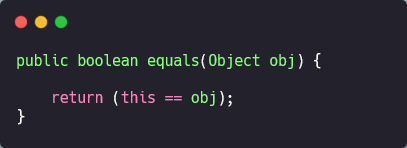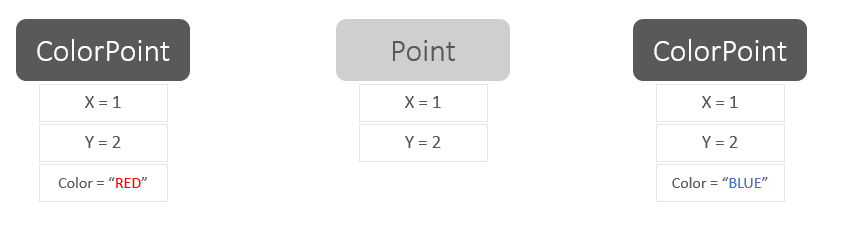# Java | Overriding equal() MethodOverriding common methods (equals, hashCode, toString, and clone) of the Object class and Comparable.compareTo() method, should be done according to the general contracts provided in the documentation to ensure proper behaviour of these methods.

## Overriding Object Common Methods

• `Object` is the super type for all classes.
• All classes inherit the default behaviour of `Object` methods (equals, hashCode, toString, and clone)
``````public boolean equals(Object obj)
public int hashCode()
public String toString()
protected Object clone() throws CloneNotSupportedException
``````
• However, if you decide to override one of these methods, then there are general contracts or instructions you should follow to ensure proper execution of these methods.

## Overriding equals(Object)

### Default Implementation

• The default behaviour of equals(Object) method is to compare objects references “identity”.
``````public boolean equals(Object obj) {

return (this == obj);
}
``````
• So, if a class creates a single instance “singleton” or unique instances, then it is better to keep default implementation.
• If we need to compare instances of a class based on their values “logical equality”, then overring equals(Object) is necessary.

### General Contracts

The equals method implements an equivalence relation on non-null object references:

• It is reflexive: for any non-null reference value x, x.equals(x) should return true.
• It is symmetric: for any non-null reference values x and y, x.equals(y) should return true if and only if y.equals(x) returns true.
• It is transitive: for any non-null reference values x, y, and z, if x.equals(y) returns true and y.equals(z) returns true, then x.equals(z) should return true.
• It is consistent: for any non-null reference values x and y, multiple invocations of x.equals(y) consistently return true or consistently return false, provided no information used in equals comparisons on the objects is modified.
• For any non-null reference value x, x.equals(null) should return false.

Reflexive

``````myObject.equals(myObject) // should return true
``````

Symmetry

``````myObject.equals(anotherObject) // returns true
anotherObject.equals(myObject) // should return true too!
``````

We can demonstrate how to get this one wrong in the following example

• `MyString` class overrides equals(Object). The method was overridden to handle passed object of type `String` which works fine. However, `String` class was NOT implemented to handle an object of type `MyString`! This implementation is violating symmetry.
``````public class MyString {

private final String s;

public MyString(String s) {
this.s = (s == null? "":s);
}

@Override
public boolean equals(Object obj) {
if(obj instanceof MyString)
return ((MyString)(obj)).s.equals(this.s);
else if(obj instanceof String)
return obj.equals(this.s);
else
return false;
}
}
``````
``````public static void main(String[] args) {

MyString myString = new MyString("cool!");
String string = "cool!";

System.out.println(myString.equals(string)); // returns true
System.out.println(string.equals(myString)); // returns false

}
``````
• `MyString` class should not accept object of type other than `MyString`.
``````@Override
public boolean equals(Object obj) {
if(obj instanceof MyString)
return ((MyString)(obj)).s.equals(this.s);
else
return false;
}
``````
``````public static void main(String[] args) {

MyString myString = new MyString("cool!");
String string = "cool!";

System.out.println(myString.equals(string)); // returns false
System.out.println(string.equals(myString)); // returns false

}
``````

Transitivity

``````firstObject.equals(secondObject) // returns true
secondObject.equals(thirdObject) // returns true
firstObject.equals(thirdObject) // must return true
``````

We can demonstrate how to get this one wrong in the following example

• We have a class `Point` with two parameters and overrides equals() method with no violation of general contracts.
• `ColorPoint` is a subclass with one extra color parameter and also overrides equals() method that includes the color paramter in the logic of equality.
``````public class Point {
private final int x;
private final int y;

public Point(int x, int y) {
this.x = x;
this.y = y;
}

@Override public boolean equals(Object obj) {
if (!(obj instanceof Point))
return false;

Point p = (Point)obj;
return p.x == x && p.y == y;
}
}
``````
``````public class ColorPoint extends Point {

private final String color;

public ColorPoint(int x, int y, String color) {
super(x, y);
this.color = color;
}

@Override public boolean equals(Object obj) {
if (!(obj instanceof ColorPoint))
return false;
return super.equals(obj) && ((ColorPoint) obj).color.equals(this.color);
}

}
``````
• `ColorPoint` equals() method violates symmetry!
``````public static void main(String[] args) {

Point point = new Point(1, 2);
ColorPoint colorPoint = new ColorPoint(1, 2, "RED");

System.out.println(point.equals(colorPoint)); // true
System.out.println(colorPoint.equals(point)); // false

}
``````
• To fix the symmetry issue we modify the equal() method as following
``````@Override
public boolean equals(Object obj) {
if (!(obj instanceof Point))
return false;

// if obj is Point but not ColorPoint
if (!(obj instanceof ColorPoint))
return obj.equals(this);

// if obj is a ColorPoint; do a full comparison
return super.equals(obj) && ((ColorPoint) obj).color.equals(this.color);
}
``````
``````public static void main(String[] args) {

Point point = new Point(1, 2);
ColorPoint colorPoint = new ColorPoint(1, 2, "RED");

System.out.println(point.equals(colorPoint)); // true
System.out.println(colorPoint.equals(point)); // true

}
``````
• However, this modified version of equals() method violates transitivity!``````public static void main(String[] args) {

ColorPoint redPoint = new ColorPoint(1, 2, "RED");
Point point = new Point(1, 2);
ColorPoint bluePoint = new ColorPoint(1, 2, "BLUE");

System.out.println(redPoint.equals(point)); // true
System.out.println(point.equals(bluePoint)); // true
System.out.println(redPoint.equals(bluePoint)); // false
}
``````
• It turns out that this is a fundamental problem in object-oriented languages.

“There is no way to extend an instantiable class and add a value component while preserving the equals contract” ~ Joshua Block’s, Effective Java

• The solution for this problem is to use composition instead of inheritance
``````public class ColorPoint  {

private final Point point;
private final String color;

public ColorPoint(Point point, String color) {
this.point = point;
this.color = color;
}

@Override
public boolean equals(Object obj) {

if (!(obj instanceof ColorPoint))
return false;

ColorPoint cp = (ColorPoint) obj;
return cp.point.equals(this.point) && cp.color.equals(this.color);
}

}
``````
``````public static void main(String[] args) {

ColorPoint redPoint = new ColorPoint(new Point(1, 2), "RED");
Point point = new Point(1, 2);
ColorPoint bluePoint = new ColorPoint(new Point(1, 2), "BLUE");

System.out.println(redPoint.equals(point)); // false
System.out.println(point.equals(bluePoint)); // false
System.out.println(redPoint.equals(bluePoint)); // false
}
``````

Consistency

• Calling equals() method on two objects should consistently return the same result until one or both of them is modified.
• This can be achieved by making the object immutable.